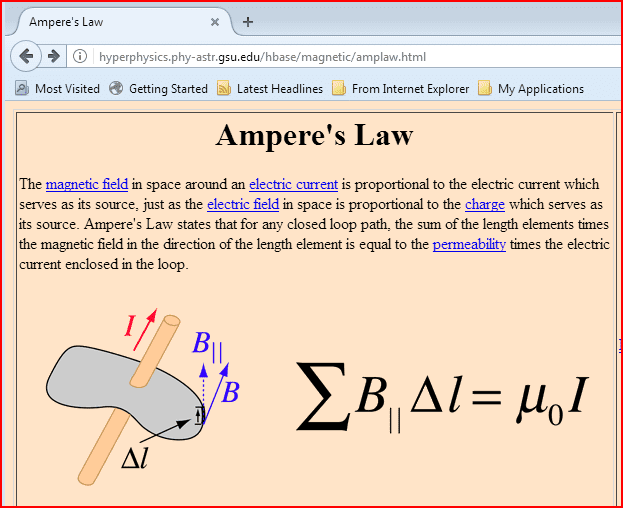# Amperian loop-conceptual question

Gold Member

## Homework Statement

I've just been introduced to Ampere's circuital Law to calculate magnetic field. I saw in a derivation for the magnetic field inside hollow straight current carrying conductor that the integral of B.dl was zero because no current was passing through the surface of the loop. This was used to imply B=0.
However, it is mentioned ∫B.dl=0 does not mean B=0 at every point on the loop. I'm confused since ∫dl (length of loop) cannot be zero therefore B has to be zero. Where am I wrong?

∫B.dl=μI(net)

## The Attempt at a Solution

Relevant details described above

Mentor
It's a vector equation. What happens when B points different ways in different parts of the path integral?Gold Member
It's a vector equation. What happens when B points different ways in different parts of the path integral?I'm finding this hard to visualize. (Since I haven't dealt with a path integral before). From what I understand we take B at every point (dl) and take its dot product at that point and summing this up across the loop gives us the path integral. Even in the case of a straight wire B points different ways as you mentioned, I'm not sure what that implies...
Is there a specific example or explanation which can make this clearer?

Mentor
Is there a specific example or explanation which can make this clearer?
Take the case of a closed path enclosing a single wire carrying a current I. Then the quantity B⋅dl will have the same sign all the way around the loop, and the path integral will give a non-zero result. But if you have two wires enclosed by that path, and the wires carry equal and opposite currents, what happens to the overall path integral?#### Attachments

Gold Member
Take the case of a closed path enclosing a single wire carrying a current I. Then the quantity B⋅dl will have the same sign all the way around the loop, and the path integral will give a non-zero result. But if you have two wires enclosed by that path, and the wires carry equal and opposite currents, what happens to the overall path integral?

View attachment 231104
Ah! the net current through the surface enclosed by the loop is zero when there are two wires carrying equal and opposite currents which gives ∫B.dl=0 bu there's still a magnetic field everywhere on the surface.
So am I correct in saying that the only thing we can say with certainty when ∫B.dl is 0 is that the net current through the surface is zero?

Gold Member
I saw in a derivation for the magnetic field inside hollow straight current carrying conductor that the integral of B.dl was zero because no current was passing through the surface of the loop. This was used to imply B=0.
What is the condition required to be able to say B=0 then?

Mentor
Ah! the net current through the surface enclosed by the loop is zero when there are two wires carrying equal and opposite currents which gives ∫B.dl=0 bu there's still a magnetic field everywhere on the surface.
So am I correct in saying that the only thing we can say with certainty when ∫B.dl is 0 is that the net current through the surface is zero?
Correct. There are B components pointing in opposite directions around the circumference of the loop, and in total they sum to zero. But at any particular point along that path, the sum of the B components from the two wires may not necessarily be zero.

Mentor
What is the condition required to be able to say B=0 then?
In general that requires no source currents nearby (an no moving charges nearby).

Gold Member
In general that requires no source currents nearby (an no moving charges nearby).
This is the part where I'm confused,
what is the difference between the case when there are two wires carrying current in opposite directions and in case 2 when we're talking about the inside of a hollow straight current carrying conductor.My textbooks says for case 2 I(enclosed)=0, so ∫B.dl=0⇒B∫dl=0⇒B=0 while its clearly not so in case 1.

Mentor
Can you Upload a diagram for case 2? B can only be taken out of the integral if it does not depend on the position along the path. So unless B(l)=constant all along the path, it needs to be part of the integration.